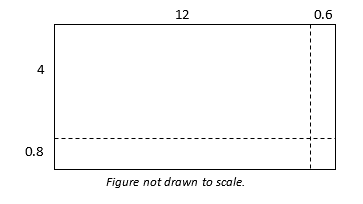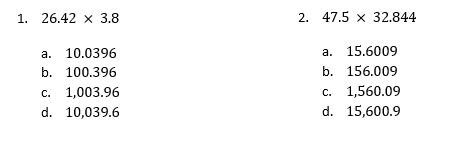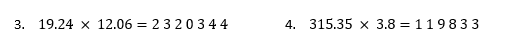Match Fishtank is now Fishtank Learning!

# Multi-Digit and Fraction Computation

## Objective

Multiply decimals using strategies, and develop an understanding of the standard algorithm.

## Common Core Standards

### Core Standards

?

• 6.NS.B.3 — Fluently add, subtract, multiply, and divide multi-digit decimals using the standard algorithm for each operation.

?

• 5.NBT.A.2

• 5.NBT.B.5

• 5.NBT.B.7

## Criteria for Success

?

1. Solve decimal multiplication problems using partial products.
2. Use estimation to estimate and understand products of decimals.
3. Understand the relationship between a whole number multiplication problem and a decimal multiplication problem with the same digits; develop an understanding of the standard algorithm for multiplying decimals.
4. Multiply decimals using the standard algorithm.

## Tips for Teachers

?

• In fifth grade, students learn different strategies to multiply decimals. In this lesson, they review some of those strategies and are guided toward understanding the standard algorithm as an efficient way to compute. Lesson 9 continues this work with more practice and fluency development.
• The following tools or materials are useful for this lesson: large graph or grid paper for computations, calculators to check answers.

#### Remote Learning Guidance

If you need to adapt or shorten this lesson for remote learning, we suggest prioritizing Anchor Problem 2 or 3 (benefit from worked examples) and Anchor Problem 4 (can be done independently). Find more guidance on adapting our math curriculum for remote learning here.

#### Fishtank Plus

• Problem Set
• Student Handout Editor
• Vocabulary Package

## Anchor Problems

?

### Problem 1

Use partial products to calculate the product of ${12.6 × 4.8}$.### Problem 2

For the two problems below, use estimation to determine the product without calculating it exactly.For the two problems below, use estimation to determine where the decimal point is located in each product.### Problem 3

Consider the multiplication problem: ${24 × 3=72}$.

Now consider the problem: ${24 × 0.3}$.

Using properties of operations, it can be written as: ${24 \times (3 \times 0.1) = (24 × 3) \times 0.1=7.2}$

Rewrite the following multiplication problems like the one above to determine each product.

• ${2.4 \times 3}$
• ${24 \times 0.03}$
• ${2.4 \times 0.3}$

Describe what you notice. What is the relationship between a whole number multiplication problem and a decimal multiplication problem with the same digits? Why does this make sense?

### Problem 4

Given the multiplication problem ${{52.83 \times 2.6}}$

1. Determine an estimate.
2. Find the related product of ${5283 \times 26}$.
3. Determine the product of ${{52.83 \times 2.6}}$.

## Problem Set

?The following resources include problems and activities aligned to the objective of the lesson that can be used to create your own problem set.

?

Given the multiplication problem: ${9.36 × 4.22 }$

1. Determine an estimate.
2. Find the related product of ${936 \times 422}$.
3. Determine the product of ${9.36 \times 4.22}$.

?